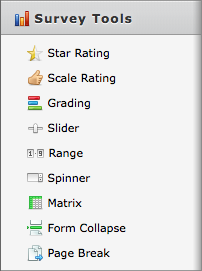# How to add a formula to a field which calculates the total and/or percentage of those values to be automatically posted in another field.

•noycenortheast
Asked on April 03, 2012 at 02:59 PM

If I am collecting numbers from various fields on a form, I would like to create a field which is automatically updated based on the values in those fields.

For example

InputField1 = 5

InputField2 = 4

InputField3 = 4

OutputField1 = InputField1 + InnputField2 + InputField3

OutputField2 = OutputField1 divided-by 100

What is needed is some basic calculations that you can find on any spreadsheet

•jeanettebmz
Answered on April 03, 2012 at 05:38 PM

Currently, our forms are not able to calculate and show percentages, however , you might use the Matrix form , which is under the  Survey Tools, which allows you to get the total from previous input fieldsHope this sorts out your question. Should you need more assistance, let us know

Jeanette

•gorlando
Answered on May 16, 2012 at 11:28 AM

can i get more details on this?

what does this mean?

"use the Matrix form , which is under the  Survey Tools, which allows you to get the total from previous input fields"

•jeanettebmz
Answered on May 16, 2012 at 04:26 PM

@gorlando

I apologize for the mistake, I was actually talking about the grading tool

Best regards

Jeanette

•cchl
Answered on August 07, 2014 at 12:08 PM

I need a way to calculate the sum of the columns in a matrix. I can't figure out the matrix calculation tool. Is there a tutorial for it?